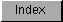```
These  counters  will "wrap around" in either the positive or negative
direction. If you wish to use the mouse to control something that is
happening on the screen, you must read the  counters  at least once each
vertical blanking  period and save the previous contents of the
registers. Then you can subtract from the previous readings to determine
direction of movement and speed.

The mouse produces about 200 count pulses per inch of movement in either a
horizontal or vertical direction.  Vertical blanking  happens once each
1/60th of a second. If you read the mouse once each  vertical blanking
period, you will most likely find a count difference (from the previous
count) of less than 127. Only if a user moves the mouse at a speed of more
than 38 inches per second will the  counter  values wrap.  Fast-action
games may need to read the mouse register twice per frame to prevent
counter  overrun.

If you subtract the current count from the previous count, the absolute
value of the difference will represent the speed. The sign of the
difference (positive or negative) lets you determine which direction the
mouse is traveling.

The easiest way to calculate mouse velocity is with 8-bit signed
arithmetic. The new value of a  counter  minus the previous value will
represent the number of mouse counts since the last check. The example
shown in Table 8-2 presents an alternate method.  It treats both counts as
unsigned values, ranging from 0 to 255.  A count of 100 pulses is measured
in each case.

Table 8-2: Determining the Direction of the Mouse

Previous    Current
Count       Count     Direction
--------    -------    ---------
200         100      Up (Left)
100         200     Down (Right)
200          45       Down *
45         200        Up **

Notes for Table 8-2:

*  Because 200-45 = 155, which is more than 127, the true count must
be 255 - ( 200-45) = 100; the direction is down.

** 45 - 200 = -155.  Because the absolute value of -155 exceeds 127,
the true count must be  255 + (-155) = 100; the direction is up.

```